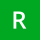Home Communities
IT Knowledge
Inspiration
Languages
EN

# JavaScript - moving average

11 points
Created by:Reilly-Collier
710

In this article, we would like to show how to calculate the moving average in JavaScript

Quick solution:

``````// ONLINE-RUNNER:browser;

const calculateItemsSum = (data, start, stop) => {
let sum = 0;
for (let j = start; j < stop; ++j) {
sum += data[j];
}
return sum;
};

const caculateMovingAverage = (data, window) => {
const steps = data.length - window;
const result = [ ];
for (let i = 0; i < steps; ++i) {
const sum = calculateItemsSum(data, i, i + window);
result.push(sum / window);
}
return result;
};

// Usage example:

const data = [1, 2, 3, 4, 5, 6];
const average = caculateMovingAverage(data, 3);

console.log(average);  // [2, 3, 4]``````

Where:

• `window` means a number of elements used to calculate the average during one step.

Note: the above solution has O(n^2) complexity.

## Optimal solution example

The presented solution in this section has only O(n) complexity.

``````// ONLINE-RUNNER:browser;

function caculateMovingAverage(data, window) {
var result = [ ];
if (data.length < window) {
return result;
}
var sum = 0;
for (var i = 0; i < window; ++i) {
sum += data[i];
}
result.push(sum / window);
var steps = data.length - window - 1;
for (var i = 0; i < steps; ++i) {
sum -= data[i];
sum += data[i + window];
result.push(sum / window);
}
return result;
}

// Usage example:

var data = [1, 2, 3, 4, 5, 6];
var average = caculateMovingAverage(data, 3)

console.log(average);  // [2, 3, 4]``````

Warning: the above solution uses `-=` and `+=` operations on the `sum` variable that makes numerical mistakes when we work on floating numbers - it is good to modify below source code and recompute `sum` once per some amount of iterations (amount may be selectes empiricaly).

## References

Join to our subscribers to be up to date with content, news and offers.